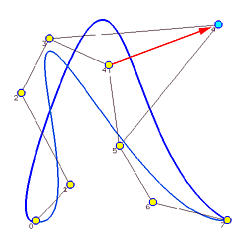# Curve Global ApproximationIn interpolation, the interpolating curve passes through all given data points in the given order. As discussed on the Global Interpolation page, an interpolating curve may wiggle through all data points rather than following the data polygon closely. The approximation technique is introduced to overcome this problem by relaxing the strict requirement that the curve must contain all data points. In global approximation, except for the first and last data points, the curve does not have to contain every point.

To measure how well a curve can "approximate" the given data polygon, the concept of error distance is used. The error distance is the distance between a data point and its "corresponding" point on the curve. Thus, if the sum of these error distances is minimized, the curve should follow the shape of the data polygon closely. An interpolating curve is certainly such a solution because the error distance of each data point is zero. However, the formulation to be discussed is unlikely to yield an interpolating curve. A curve obtained this way is referred to as an approximation curve.

Suppose we are given n+1 data points D0, D1, ..., Dn, and wish to find a B-spline curve that follows the shape of the data polygon without actually containing the data points. To do so, we need two more input: the number of control points (i.e., h+1) and a degree (p), where n > h >= p >= 1 must hold. Thus, approximation is more flexible than interpolation, because we not only select a degree but also the number of control points. The following is a summary of our problem:

### Global Curve Approximation

Given a set of n+1 data points, D0, D1, ..., Dn, a degree p, and a number h, where n > h >= p >= 1, find a B-spline curve of degree p defined by h+1 control points that satisfies the following conditions:
1. this curve contains the first and last data points (i.e., D0 and Dn) and
2. this curve approximates the data polygon in the sense of least square.

With h and p in hand, we can determine a set of parameters and a knot vector. Let the parameters be t0, t1, ..., tn. Note that the number of parameters is equal to the number of data points. Now, suppose the approximation B-spline of degree p is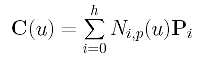where P0, P1, ..., Ph are the h+1 unknown control points. Since we want the curve to pass the first and last data points, we have D0 = C(0) = P0 and Dn = C(1) = Ph. Therefore, there are only h - 1 unknown control points P1, P2, ..., Ph-1. Taking this into consideration, the curve equation becomes the following: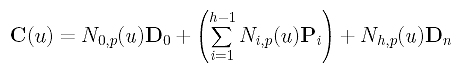### The Meaning of Least Square

How do we measure the error distance? Because parameter tk corresponds to data point Dk, the distance between Dk and the corresponding point of tk on the curve is |Dk - C(tk)|. Since this distance consists of a square root which is not easy to handle, we choose to use the squared distance |Dk - C(tk)|2. Hence, the sum of all squared error distances is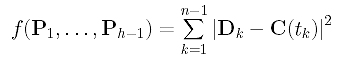Our goal is, of course, to find those control points P1, ..., Ph-1 such that the function f() is minimized. Thus, the approximation is done in the sense of least square.

### Finding a Solution

This section is a little messy and requires some knowledge in linear algebra. First, let us rewrite Dk - C(tk) into a different form: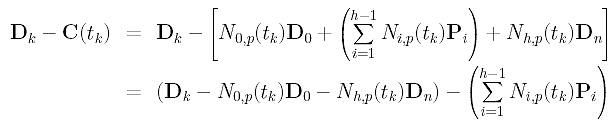In the above, D0, Dk and Dn are given, and N0,p(tk) and Nh,p(tk) can be obtained by evaluating N0,p(u) and Nh,p(u) at tk. Click here to learn how to compute these coefficients. For convenience, let us define a new vector Qk as: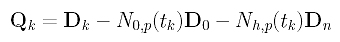Then, the sum-of-square function f() can be written as follows: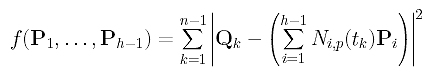Next, we shall find out what the squared error distance looks like. Recall the identity x.x = |x|2. This means the inner product of vector x with itself gives the squared length of x. Thus, the error square term can be rewritten as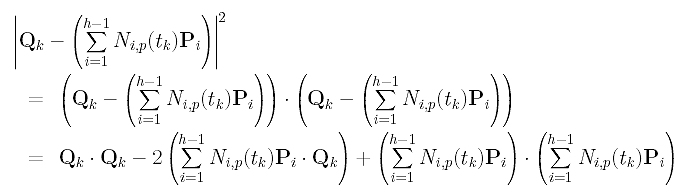Then, function f() becomes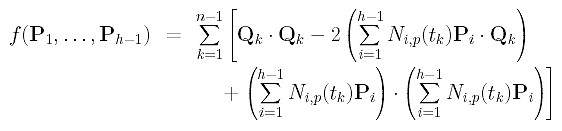How do we minimize this function? Function f() is actually an elliptic paraboloid in variables P1, ..., Ph-1. Therefore, we differentiate f() with respect to each Pg and find the common zeros of these partial derivatives. These zeros are the values at which function f() attends its minimum.

In computing the derivative with respect to Pg, note that all Qk's and Ni,p(tk) are constants (i.e., no Pk involved) and their partial derivatives with respect any Pg must be zero. Therefore, we have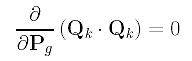Consider the second term in the summation, which is the sum of Ni,p(tk)Pi .Qk's. The derivative of each sub-term is computed as follows: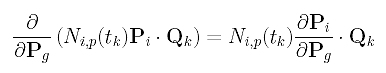The partial derivative of Pi with respect to Pg is non-zero only if i = g. Therefore, the partial derivative of the second term is the following: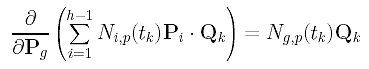The derivative of the third term is more complicated; but it is still simple. The following uses the multiplication rule (f.g)' = f'.g + f.g'.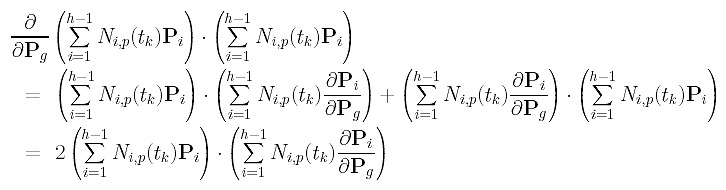Since the partial derivative of Pi with respect to Pg is zero if i is not equal to g, the partial derivative of the third term in the summation with respect to Pg is: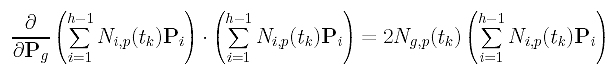Combining these results, the partial derivative of f() with respect to Pg is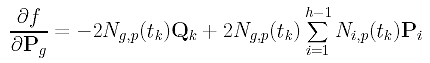Setting it to zero, we have the following: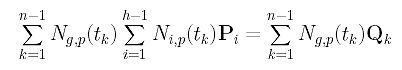Since we have h-1 variables, g runs from 1 to h-1 and there are h-1 such equations. Note that these equations are linear in the unknowns Pi's. Before we go on, let us define three matrices: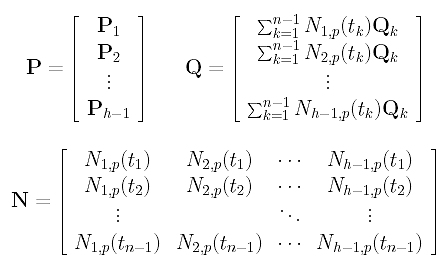Here, the k-th row of P is the vector Pk, the k-th row of Q is the right-hand side of the k-th equation above, and the k-th row of N contains the values of evaluating N1,p(u), N2,p(u), ..., Nh-1,p(u) at tk. Therefore, if the input data points are s-dimensional vectors, P, N and Q are (h-1)×s, (n-1)×(h-1) and (h-1)×s matrices, respectively.

Now, let us rewrite the g-th linear equationinto a different form so that the coefficient of Pi can easily be read off: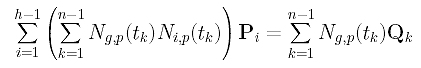Finally, the coefficient of Pi is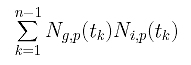If you look at matrix N, you should see that Ng,p(t1), Ng,p(t2), ..., Ng,p(tn-1) are the g-th column of N, and Ni,p(t1), Ni,p(t2), ..., Ni,p(tn-1) are the i-th column of N. Note that the g-th column of N is the g-th row of N's transpose matrix NT, and the coefficient of Pi is the "inner" product of the g-th row of NT and the i-th column of N. With this observation, the system of linear equations can be rewritten as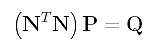Since N and Q are known, solving this system of linear equations for P yields the desired control points. And, of course, we have found the solution.

### An Algorithm

Although the development in the previous section is lengthy and tedious, the final result is surprisingly simple: solving a system of linear equations just like we encountered in global interpolation. As a result, the algorithm for global approximation is also quite simple.

Input: n+1 data points D0, D1, ..., Dn, a degree p, and the desired number of control points h+1;
Output: A B-spline curve of degree p, defined by h+1 control points, that approximates the given data points;
Algorithm:
Obtain a set of parameters t0, ..., tn and a knot vector U;
Let P0 = D0 and Ph = Dn;
for k := 1 to n-1 do
Compute Qk with the following formula:for i := 1 to h-1 do
Compute the following and save it to the i-th row of matrix Q;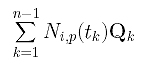/* matrix Q is available */
for k := 1 to n-1 do
for i := 1 to h-1 do
Compute Ni,p(tk) and save to row k and column i of N;
/* matrix N is available */
Compute M = NT.N;
Solving for P from M.P = Q;
Row i of P is control point Pi;
Control points P0, ..., Ph, knot vector U and degree p determines an approximation B-spline curve;

### The Impact of Degree and Number of Control Points

It is obvious that the data points affect the shape of the approximation curve. What is the impact of degree p and the number of control points on the shape of the curve? The following figures show some approximation curves for 10 data points (n=9) with various degrees and numbers of control points. Each row gives the approximation curves of the same degree but with different number of control points, while each column shows the same number of control points with different degree. Note that all curves use the centripetal method for computing its parameters.

 Points = 4 Points = 5 Points = 6 Points = 7 Deg 2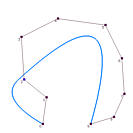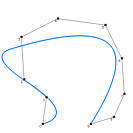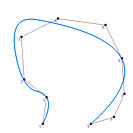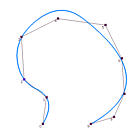Deg 3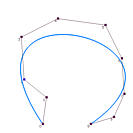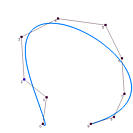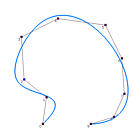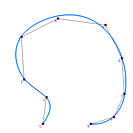Deg 4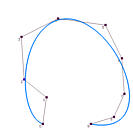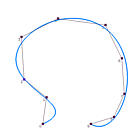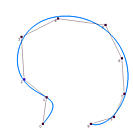Deg 5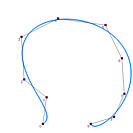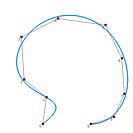It is understandable that lower degree curves in general will not be able to approximate the data polygon well because they are not very flexible. Therefore, on each column, higher degree curves yield better results (i.e., closer to the data polygon). By the same reason, more control points offer higher flexibility of the approximation curve. Hence, on each row, as the number of control points increases, the curve becomes closer to the data polygon.

Shall we use higher degree curves and many control points? The answer is no because global approximation may use fewer number of control points than global interpolation. If the number of control points is equal to the number of data points, global approximation becomes global interpolation, and we can just use global interpolation! As for degree, we certainly want the smallest one as long as the produced curve can capture the shape of the data polygon.

### Why Is This Method Global?

This approximation method is global because changing the position of a data point causes the entire curve to change. The yellow dots in the following image are the given data points to be approximated by a B-spline curve of degree 3 and 5 control points (i.e., n = 7, p=3 and h = 4). The parameters are computed with the centripetal method. Suppose data point 4 is moved to a new position indicated by a light blue dot. The new approximation B-spline is the one in blue color. As you can see, except for the first and last data points which are fixed by the algorithm, the shapes of the original curve and the new one are very different. Therefore, changes due to modifying data points are global!